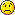^ 回到顶部
• 人生没有定律，每个人都有自己的节奏
• 本站wordpress建站教程均通过实践后发布，希望对你有帮助，如果有代码出错，请联系站长解决
• 希望你的坚持，都是因为热爱，而不是因为不甘心
• 8年wordpress建站经验，5星服务品质
• 那些不愿意让你吃亏的人，才是真正值得你深交的人，也是值得你付出时间的人
• 阿里云ECS服务器

# 零起点php入门第11课-php函数（2）

1、变量的作用域

```<?php
\$m=2;			//函数内部定义并初始化两个变量
\$n=3;
return \$m+\$n;
}
\$m=12;			//函数外部定义两个同名变量
\$n=8;
echo '<br />函数外部输出结果：'.(\$m+\$n);
?>```

```<?php
function print_num(){			//定义函数
\$x=6;			//在函数中定义变量
}
print_num();		//调用函数
echo \$x;
?>```

（代码在运行后输出了一个变量为定义的提示，这就证明了在函数外部不能够读取到函数内部定义的变量。）

2、全局变量

global 变量 1,变量 2,变量 3…

```<?php
function test(){		//定义函数
global \$a;		//声明全局变量
echo \$a;
}
\$a=7;
test();			//调用函数
?>```

3、静态变量

```<?php
function test(){		//定义函数
\$a=0;			//定义变量
\$a++;			//变量递增
echo \$a;		//输出变量值
}
test();			//第一次调用函数
test();			//第二次调用函数
test();			//第三次调用函数
?>```

static 变量名=初始值;

```<?php
function test(){		//定义函数
static \$a=0;			//定义静态变量
\$a++;			//变量递增
echo \$a;		//输出变量值
}
test();			//第一次调用函数
test();			//第二次调用函数
test();			//第三次调用函数
?>```

4、函数的其他使用方法

（1）可变函数

\$variable()

```<?php
function hello(){			//定义函数
echo '<br />Hello!<br />';
}
function hellophp(){		//定义函数
echo '<br />Hello PHP!<br />';
}
function good(){			//定义函数
echo '<br />PHP is very good!<br />';
}
\$func='good';			//定义变量 func
\$func2='hello';			//定义变量 func2
\$func();			//变量作为函数名 good()
\$func2();			//变量作为函数名 hello()
\$func='hellophp';		//对变量 func 重新赋值
\$func();		//变量作为函数名 hellophp()
?>```

PHP is very good!

Hello!

Hello PHP!

（2）函数的引用返回

function &函数名(参数列表){

return 返回值;
}

```<?php
function &test(){		//定义函数
static \$a=0;			//定义变量
\$a++;			//变量递增
return \$a;		//返回变量值
}
\$quote=&test();			//建立联系
echo "\\$a=\$quote";			//输出函数中变量 a 的值
test();			//调用函数
test();			//调用函数
echo "<br />\\$a=\$quote";			//输出函数中变量 a 的值
\$quote=15;			//改变返回值\$a 的值
test();			//调用函数
echo "<br />\\$a=\$quote";			//输出函数中变量 a 的值
?>```

\$a=1
\$a=3
\$a=16

（3）函数的递归调用

```function func_name(…){
…;
func_name(…);
…;
}
```

```<?php
function cell(\$start,\$end){			//定义细胞函数，参数 start 为开始时间，end 为结束时间
global \$sum;
for(\$i=\$start+1;\$i<\$end;\$i=\$i+2)
if(\$i+2<=\$end){
cell(\$i+2,\$end);		//递归调用
\$sum=\$sum+1;
}
}
\$sum=1;
cell(-1,35);		//由于开始时候是有一个成熟的细胞，因此需要将时间提前 1 分钟
echo "15 分钟后为\$sum 个细胞。";
?>```

（4）匿名函数

function (参数列表){

}

\$varable = function (参数列表){

}

```<?php
\$func=function (\$x,\$y){			//匿名函数与变量绑定
return \$x+\$y;
};
echo '5+6='.\$func(5,6);			//使用匿名函数
echo '<br />15+16='.\$func(15,16);
?>```

5+6=11
15+16=31### 如有疑问，请前往问答中心反馈！

1.麦谦生博客
谢谢分享
2.主宰者博客
糟了，我完全看不懂怎么办• 陌小雨网站管理员
@主宰者博客 从最最基础的开始看，很快就懂啦3.星空
这是在写php吗？
• 陌小雨网站管理员
@星空 是的，在苦学php